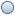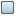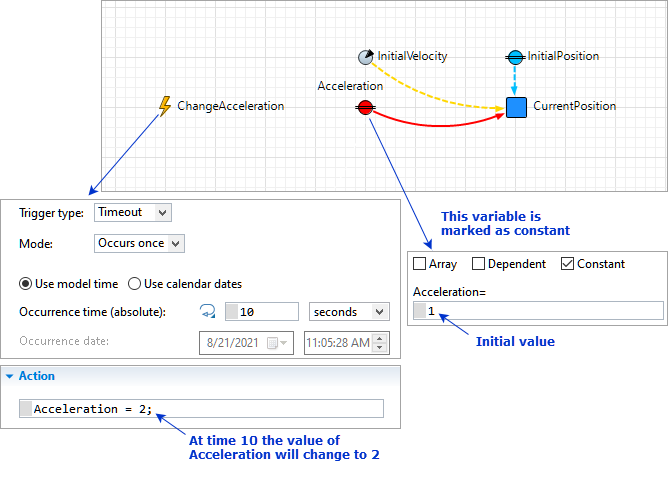AnyLogic

# Changing values of flows and dynamic variablesdynamic variables values are constantly re-evaluated according to the equations you define for them.

You can, however, change the value of such a variable by explicitly assigning a new value.

Any dynamic variable (exceptstock) can be declared constant by selecting its Constant property. The expression typed in its value field will be treated as an initial value, and will not be evaluated on each numeric integration step.

To make a variable "constant"

1. Select the variable (flow, or dynamic variable).
2. In the Properties, select the Constant checkbox.
3. You will see that the variable icon has changed (the "equal sign" icon modifier was added).

Having declared a variable as a constant, you can change the variable’s value by assigning a new value: variable = <new value>, e.g. PollutionFlow = 200;

In the figure below, the variable Acceleration is marked as constant. Its initial value is 1. At time 10 the event ChangeAcceleration will occur and change the value of Acceleration to 2.